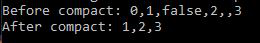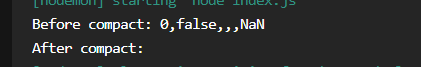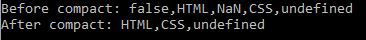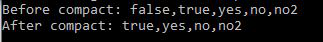# Lodash _.compact() Function

Lodash _.compact() function is used to create an array with all false values removed in JavaScript. so it returns a new array of filtered values.

### Syntax:

`_.compact(array);`

### Parameters:

• array: It is an array to be compacted.

Note: The values are false, null, 0, “”, undefined, and NaN are falsey.

### Return Value:

This function returns the array after filtering the values.

Example 1: In this example, we are passing a list of both the true and the false elements to the _.compact() function.

## javascript

 `// Requiring the lodash library ` `let _ = require(``"lodash"``); ` `  `  `// Original array to be compacted ` `let array = [0, 1, ``false``, 2, ``''``, 3]; ` `  `  `let newArray = _.compact(array); ` `console.log(``"Before compact: "` `+ array); ` `  `  `// Printing newArray  ` `console.log(``"After compact: "` `+ newArray);`

Output:Example 2: In this example, we are passing a list containing all the false values to the _.compact() function.

## javascript

 `// Requiring the lodash library ` `let _ = require(``"lodash"``); ` `  `  `// Original array to be compacted ` `let array = [0, ``false``, ``''``, undefined, NaN]; ` `  `  `let newArray = _.compact(array); ` `console.log(``"Before compact: "` `+ array); ` `  `  `// Printing newArray  ` `console.log(``"After compact: "` `+ newArray);`

Output:output

Example 3: In this example, we are passing a list which contains a false element into _.compact() function.

## javascript

 `// Requiring the lodash library ` `let _ = require(``"lodash"``);`   `// Original array to be compacted ` `let array = [``false``, ``'HTML'``, NaN,` `    ``'CSS'``, ``'undefined'``];`   `let newArray = _.compact(array);` `console.log(``"Before compact: "` `+ array);`   `// Printing newArray  ` `console.log(``"After compact: "` `+ newArray);`

Output:Example 4: In this example, we are passing a list containing modified false values to the _.reduce() function.

## javascript

 `// Requiring the lodash library ` `let _ = require(``"lodash"``); ` `  `  `// Original array to be compacted ` `let array = [``false``, ``true``, ``'yes'``, ``'no'``, ``"no2"``]; ` `  `  `let newArray = _.compact(array); ` `console.log(``"Before compact: "` `+ array); ` `  `  `// Printing newArray  ` `console.log(``"After compact: "` `+ newArray);`

Output:Whether you're preparing for your first job interview or aiming to upskill in this ever-evolving tech landscape, GeeksforGeeks Courses are your key to success. We provide top-quality content at affordable prices, all geared towards accelerating your growth in a time-bound manner. Join the millions we've already empowered, and we're here to do the same for you. Don't miss out - check it out now!

Previous
Next